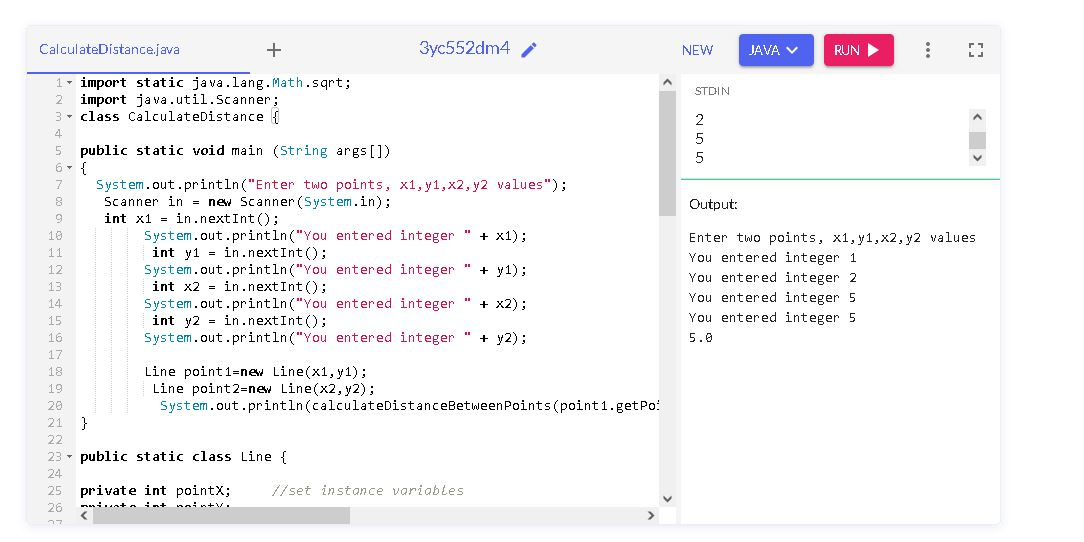# Java program to calculate distance between two points

Published August 05, 2022

In this java assignment we will write simple program to calculate distance between two points. We have two points point1 contains coordinate (x1,y1) and point2 contains coordinates (x2,y2) to calculate distance between two points we will use below Math formula

 ``Math.sqrt((y2 - y1) * (y2 - y1) + (x2 - x1) * (x2 - x1))``

In this below example we have created a Static class called Lines which contains coordinates of points.

Add one static method which will calculate distance between two points

 static public double calculateDistanceBetweenPoints(   double x1,   double y1,   double x2,   double y2) {            return Math.sqrt((y2 - y1) * (y2 - y1) + (x2 - x1) * (x2 - x1)); }

 import static java.lang.Math.sqrt; import java.util.Scanner; class CalculateDistance { public static void main (String args[]) {     System.out.println("Enter two points, x1,y1,x2,y2 values");      Scanner in = new Scanner(System.in);      int x1 = in.nextInt();         System.out.println("You entered integer " + x1);          int y1 = in.nextInt();         System.out.println("You entered integer " + y1);          int x2 = in.nextInt();         System.out.println("You entered integer " + x2);          int y2 = in.nextInt();         System.out.println("You entered integer " + y2);                  Line point1=new Line(x1,y1);          Line point2=new Line(x2,y2);             System.out.println(calculateDistanceBetweenPoints(point1.getPointX(),point1.getPointY(),point2.getPointX(),point2.getPointY())); } public static class Line { private int pointX;     //set instance variables private int pointY; public void setPoints() {       //method to set the points values     pointX = 10;     pointY = 10; } public Line(int x, int y){    //method to set arguments to instance variables     this.pointX = x;     this.pointY = y; } ///////////////////////////////////////////////// public int getPointX() {     return pointX; } public void setPointX(int x) {     this.pointX = x; } /////////////////////////////////////////////// public int getPointY() {     return pointY; } public void setPointY(int y) {     this.pointY = y; } /////////////////////////////////////////////// public String toString(){                       //toString method to display the values     return "(x,y) = (" + pointX + "," + pointY+")"; } } static public double calculateDistanceBetweenPoints(   double x1,   double y1,   double x2,   double y2) {            return Math.sqrt((y2 - y1) * (y2 - y1) + (x2 - x1) * (x2 - x1)); } }

Run the above code on Java online compilersArticle Contributed By :133 Views
Projects By category
Categories